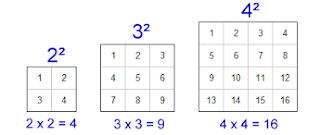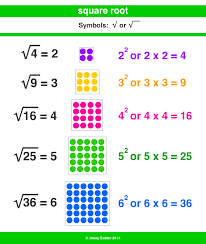## Specialist Blogs

Mme. Stella: https://stjudesfrench-stella.blogspot.com

Mr. Oliver: https://mrolivermusicclass.blogspot.com

Mr. Orr: https://tysonorr.blogspot.com

## Tuesday, 16 October 2018

### Tuesday October 16, 2018

Tremendous Tuesday!!

We began the day with math. We reviewed the homework from last night and then we moved onto learning about squaring, square numbers and square roots. First, we reviewed the important terms related to division.

Dividend:the number being divided
49÷7=7

Divisor:The number we divide by

49÷7=7

Quotient:The answer after we divide one number by another

49÷7=

Then we learned that:

A Square Number is When the quotient is equal to the divisor, the dividend is a square number
49÷7=7

Square:The square of a number is the number times itself. For example, the square of 3 is 3x3. The square of 4 is 4x4.

Squaring: When we multiply a number by itself, we square the number.
Squaring: 7x7 = 49
So 7²=49

Square rootThe square root is just the opposite of the square. You can think of it as the "root" of the square or the number that was used to make the square.

√49 = √ 7²

=7

The square root of a number is a value that, when multiplied by itself, gives the number.

Example: 4 × 4 = 16, so a square root of 16 is 4.

We watched a video to get a better understanding about squaring, square numbers and square roots:Then we did a matching activity where we needed to match the square root to the whole number or the whole number to the squared number.

After French we reviewed again about squaring, square numbers and square roots.

After first recess and lunch we learned about ‘Before Midnight Poems.’ We learned that this is a poem that uses all the senses and a time formula to describe a spooky topic. We discussed the success criteria when writing our own ‘Before Midnight Poem’ and we will write our own tomorrow.

When we returned, we continued with our Zentangle art. Then we continued building our electric cars.

After second recess and lunch we again reviewed the concepts of squaring, square numbers and square roots.

Then we continued building our electric cars. Most students are finished or nearly finished building their cars. The next step will be to test them!! Very exciting!

We had a very ‘square’ day.

Have a great night!!